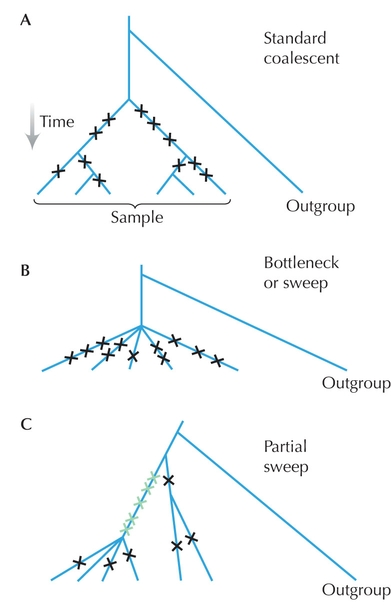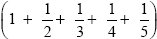Figure WN19.3. Deviations from the standard model of neutral evolution can be detected by comparing different measures of genetic diversity. In each of these diagrams, there is a sample of 6 genes, which includes 11 segregating variants (crosses). This gives an estimate of θW = 11 /= 4.82 (A) The average number of pairwise differences is θπ = 5.4. These estimates of diversity are similar; this example is typical of the standard neutral coalescent. (B) A severe bottleneck or selective sweep leads to a “star” genealogy, with an excess of rare variants. Here, θπ = 2.67, and so Tajima’s D = (θπ – θW)/s.d. is negative. (C) In this example, a partial selective sweep has raised five variants to high frequency (green). θπ = 3.6, so that Tajima’s D is weakly positive. Fay and Wu’s (2000) measure of diversity, which gives greatest weight to derived variants at high frequency, is θH = 8.93. Hence, their H statistic is strongly positive, indicating a partial selective sweep.